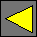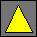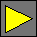April 2002 Draft JavaScript 2.0 Core Language VariablesFriday, April 12, 2002

Variable Definitions

VariableDefinition  VariableDefinitionKind VariableBindingListallowIn
VariableDefinitionKind
var
|  const
VariableBindingList
VariableBinding
|  VariableBindingList , VariableBinding
VariableBinding  TypedIdentifier VariableInitialisation
VariableInitialisation
«empty»
|  = VariableInitialiser
VariableInitialiser
AssignmentExpression
TypedIdentifier
|  Identifier : TypeExpression

A SimpleVariableDefinition represents the subset of VariableDefinition expansions that may be used when the variable definition is used as a Substatement instead of a Directive in non-strict mode. In strict mode variable definitions may not be used as substatements.

SimpleVariableDefinition  var UntypedVariableBindingList
UntypedVariableBindingList
UntypedVariableBinding  Identifier VariableInitialisationallowIn

A variable defined with var can be modified, while one defined with const is read-only after its value is set. Identifier is the name of the variable and TypeExpression is its type. Identifier can be any non-reserved identifier. TypeExpression must be a compile-time expression that evaluates to a type t other than Never. TypeExpression may contain forward references to compile-time constants defined later in the program.

If provided, AssignmentExpression gives the variable’s initial value v. If AssignmentExpression is not provided in a var definition, then undefined is assumed. If the variable being defined is not an instance member of a class, then the AssignmentExpression is evaluated at the time the variable definition is evaluated. The resulting value is then implicitly coerced to the variable’s type t and stored in the variable. If the variable is defined using var, any values subsequently assigned to the variable are also implicitly coerced to type t at the time of each such assignment. If the variable is an instance member of a class, then the AssignmentExpression is evaluated each time an instance of the class is constructed.

Reading or writing a variable before its definition is evaluated signals an error except when the variable definition has no attributes and no type and strict mode is not in effect; in that case, the variable may be read or written prior to its definition being evaluated and its initial value is undefined.

Multiple variables separated by commas can be defined in the same VariableDefinition. The values of earlier variables are available in the AssignmentExpressions of later variables.

If omitted, TypeExpression defaults to type Object. Thus, the definition

var a, b=3, c:Integer=7, d, e:Integer, f:Number=c;

is equivalent to:

var a:Object = undefined;
var b:Object = 3;
var c:Integer = 7;
var d:Object = undefined;
var e:Integer = undefined;   // Implicitly coerced to NaN
var f:Number = c;            // 7

The compiler might issue a warning for a VariableDefinition that contains an untyped variable prior to a typed variable to remind programmers that the type of d is Object rather than Integer in var d, e:Integer.

Constant Definitions

const means that assignments to Identifier are not allowed after the constant has been set. Once defined, a constant cannot be redefined in the same scope, even if the redefinition would be to the same value. If the VariableBinding in a const declaration does not contain an initializer, then the constant may be written once after it is defined. Any attempt to read the constant prior to writing its value will result in an error. For example:

function f(x) {return x+c}

f(3);           // Error: c’s value is not defined
const c = 5;
f(3);           // Returns 8
const c = 5;    // Error: redefining c

Just like any other definition, a constant may be rebound after leaving its scope. For example, the following is legal; j is local to the block, so a new j binding is created each time through the loop:

var k = 0;
for (var i = 0; i < 10; i++) {
const j = i;
k += j;
}

A const definition preceded by the compile attribute defines a compile-time constant. Such a definition is evaluated at compile time instead of run time. The initializer is required in a compile const definition and must be a compile-time expression without any forward references. The value of the constant being declared may be read just like any other variable by run-time expressions, compile-time expressions below the constant declaration, and compile-time expressions above the constant declaration if they permit forward references.

Inside a class, const preceded by final defines an instance member. Preceding it with abstract or virtual would also define an instance member, but is only useful if one wants subclasses to be able to override the constant. Precede it with static to define a global member. The default is final.

If const is declaring an instance member m of a class, then the initializer is evaluated each time an instance of the class is constructed. If absent, then the member’s property may be written exactly once, cannot be re-written after it has been written, and must be written before it can be read. For example:

class C {
static const red = 0xFF0000;      // Defines static constant C.red
static const green = 0x00FF00;    // Defines static constant C.green
static const blue = 0x0000FF;     // Defines static constant C.blue
static const infrared;            // Defines uninitialized static constant C.blue

const myColor;                    // Defines instance constant C::myColor with value given by constructor
final const yourColor;            // Defines instance constant C::yourColor with value given by constructor
const ourColor = 0;               // Defines instance constant C::ourColor that is always zero (not very useful)
virtual const theirColor = 0;     // Defines instance constant C::theirColor that can be overridden by subclasses

constructor function C(x:int32) {
myColor = x;                    // Sets this instance’s myColor
ourColor = x;                   // Error: ourColor is already set to 0
myColor = x;                    // Error: myColor can be set only once
var a = [x, this];
a.yourColor = x;             // Sets this instance’s yourColor
}
}

Getters and Setters

A definition var x:t = v internally creates a hidden variable and defines a getter and a setter to access that variable:

• Evaluate t, which should evaluate to a type.
• Create an anonymous variable .
• Implicitly coerce undefined to type t (such a coercion must exist for every type) and assign the result to .
• Define a getter function get x():t {return }.
• Define a setter function set x(a:t):Void { = a}.
• Evaluate v, implicitly coerce it to type t, and assign the result to .

A definition const x:t = v internally creates a hidden variable and defines a getter to access that variable:

• Evaluate t, which should evaluate to a type.
• Create an anonymous variable .
• Define a getter function get x():t {return }.
• Define a setter function set x(a:t):Never {throw ConstWriteError}.
• Evaluate v, implicitly coerce it to type t, and assign the result to .

This relationship between a variable and its getter and setter is normally transparent but can be exploited occasionally. For instance, a variable can be declared that is private for writing but public for reading:

private var name:String;
public export get name;

A subclass may override a variable’s getter or setter. To do this, the original variable has to be declared non-final because variables are final by default:

class C {
virtual var x:Integer;
var y:Integer;
}

class D extends C {
override function set x(a:Integer):Integer {y = a*2}
}

var c = new C;
c.x = 5;
c.x;       // Returns 5
c.y;       // Returns NaN (the default value for an Integer variable)
var d = new D;
d.x = 5;
d.x;       // Returns NaN
d.y;       // Returns 10

 Waldemar Horwat Last modified Friday, April 12, 2002